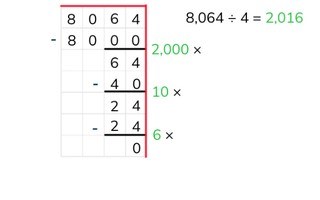Partial quotients division with a number greater than 1,000 by a number >10

# Partial quotients division with a number greater than 1,000 by a number >10

Students learn partial quotients division with a number greater than 1,000.8,000 schools use Gynzy92,000 teachers use Gynzy1,600,000 students use Gynzy

## General

The students learn partial quotients division with a number greater than 1,000, without remainder.

## Standards

CCSS.Math.Content.6.NS.B.2

## Learning objective

Students will be able to use partial quotients to divide a number greater than 1,000 without remainder.

## Introduction

On the interactive whiteboard there are a few problems, for which a child states what the answer is. The students must solve each problem on their own to determine if the answer of each child is correct or not.

## Instruction

Explain that with partial quotients division you break up the problem into steps and solve it in this way. You write down the problem and then draw a line underneath it. Next you can use a helping row from the table of the number that you are dividing by. In this row you don't have to include every problem. You can double or halve some problems, or imagine a 0 at the end. After this you solve the problem step by step to see how many times 312 fits in 8,112. After each step you write down how much you have left over. If you don't have anything left, then the problem is finished. You then add up all of the numbers that give how many times 312 fits in 8,112 (20, 5, and 1). Have the students practice in pairs. They discuss with one another how they should solve the problem using partial quotients. After that, have the students solve a few more problems using partial quotients. Ask various students what steps they took. Encourage the students to take as large steps as they are able.

Check whether the students can use partial quotients to divide by asking the following questions:
- Why is it useful to be able to divide using partial quotients?
- What aid can you use when dividing using partial quotients?
- Solve this problem using partial quotients division: 8,056 ÷ 38

## Quiz

The students test their understanding of partial quotients division with a number greater than 1,000 through ten exercises. In some of the exercises, the students must solve a division problem using partial quotients, and they are given possible answers to choose from. For other exercises, the students must produce an answer on their own. Some of the exercises are division problems with larger numbers.

## Closing

You discuss once again with the students how it is important to be able to divide using partial quotients with numbers greater than 1,000, because that is how you can solve problems in an easier way. Check if the students know that with partial quotients division they solve parts of the problem in steps and that they can use a helping row to help them with their calculations. On the interactive whiteboard there are a few problems that the students must solve using partial quotients division.

## Teaching tips

When students have difficulty with partial quotients division, you can have them make helping rows from the table of the number that they are dividing by. Have them solve the problem step by step. Check each of the steps that the students take and try to encourage them to take larger steps (not the step 10 × 3 = 30 ten times, but in one step 100 × 3 = 300).

## Instruction materials

Graphing paper

### The online teaching platform for interactive whiteboards and displays in schools

• Save time building lessons

• Manage the classroom more efficiently

• Increase student engagement